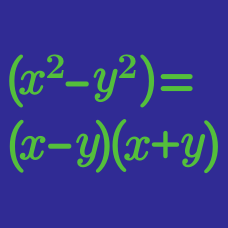Algebra

# Factoring Polynomials of Higher Degree

The polynomial $x^4-7x^3-76x^2-7x+1$ is factorized as $(x^2+ax+b)(x^2+cx+d),$ where $$a$$, $$b$$, $$c$$ and $$d$$ are real numbers and $$a>0$$. What is the value of $$ad-bc$$?

The polynomial $x^5-5x^4-31x^3-31x^2-5x+1$ is factorized as $(x+k)(x^2+ax+b)(x^2+cx+d),$ where $$a$$, $$b$$, $$c$$, $$d$$ and $$k$$ are constants and $$a>0$$. What is the value of $$(a+k)d-bc$$?

Suppose $$f(x,y)$$ and $$g(x,y)$$ are non-constant polynomials with integer coefficients in two variables, $$x$$ and $$y$$, such that $f(x,y)\cdot g(x,y)=x^3-3y^3+3x^2-xy^3$ Find $$|f(3,3)|+|g(3,3)|.$$

If $$a$$, $$b$$, $$c$$, $$d$$ and $$e$$ are non-negative integers such that

$x^6-289x^4-x^2+289=(x-a)(x+b)(x-c)(x+d)(x^2+ex+1),$

what is the value of $$a+b+c+d+e$$?

If $$a$$ and $$b$$ are positive numbers satisfying \begin{align} & x^4-5x^3+7x^2-43x+40 \\ &= (x-a)(x-b)(x^2+x+c), \end{align} what is the value of $$a+b+c$$?

×

Problem Loading...

Note Loading...

Set Loading...